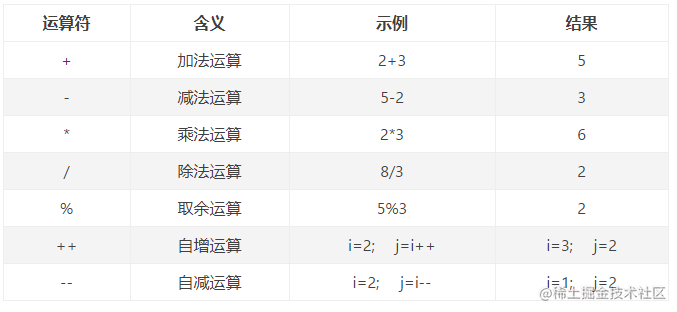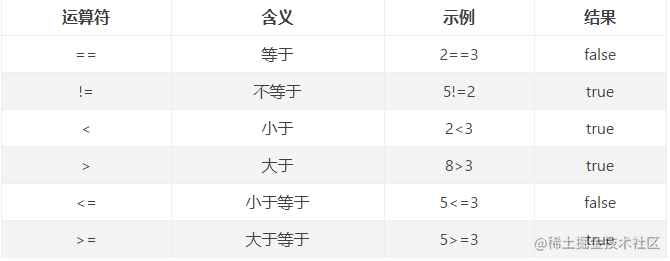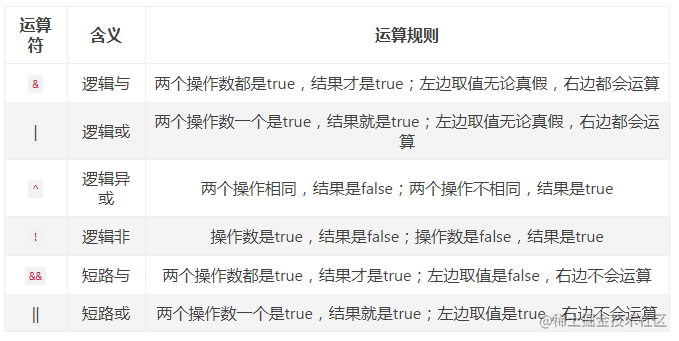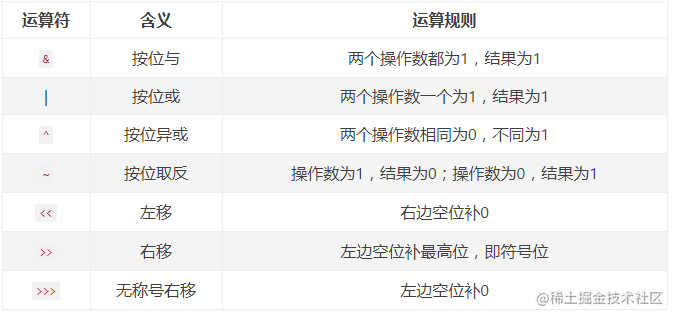# Java运算符及运算符的优先级

## 介绍

Java语言中提供了很多运算符来操作变量，现总结以下七种：

1. 赋值运算符
2. 算术运算符
3. 关系运算符
4. 逻辑运算符
5. 位运算符
6. 三目运算符
7. instanceof运算符

## 二、算术运算符• 除法运算，两个操作数是整型的，结果也会是整型的，舍弃掉小数部分；如果有一个数是浮点数，结果将自动转型为浮点型
• 取余运算，两个操作数是整型的，结果也会是整型的，如果有一个数是浮点数，结果将自动转型为浮点型
• 自增自减运算，a++ 相当于 a = a + 1, a++ 是先运用在计算，++a 先计算在运用

## 三、关系运算符• " = "是赋值运算，" == "是等于运算
• " > "、" < "、" >= "、" <= "只支持数值类型的比较，" == "、" != "支持所有数据类型的比较
• 关系表达式的运算结果是布尔值

## 四、逻辑运算符• 操作数只能是布尔型，操作结果也是布尔型
• & 和 && 的区别： && 左边是false时，不计算右边的表达式，左假即假； & 无论左边真假都会计算右边的表达式

## 五、位运算符``````a = 0011 1100 ;
b = 0000 1101 ;

a & b = 0000 1100 ;
a | b = 0011 1101 ;
a ^ b = 0011 0001 ;
~a = 1100 0011 ;
a<<2  = 1111 0000 ;
a>>2  = 1111 ;
a>>>2 = 0000 1111 ;

## 六、三目运算符

``````int m,n;
m = 5<10 ? 10 : 20;  //先判断5<7，为真，则m = 10
n = 5>10 ? 10 : 20;  //先判断5<7，为假，则n = 20

## 七、instanceof运算符

instanceof运算符用于操作对象实例，检查该对象是否是一个特定类型（类类型或接口类型），结果返回一个布尔值 示例：

``````String name = "张三";
boolean flag = name instanceof String;  //name是String类型的，返回true

## 运算符号的优先级

1( )　[ ] 　.从左到右
2! 　~　 ++　 --从右到左
3*　 /　 %从左到右
4+　 -从左到右
5<< 　>>　 >>>从左到右
6< 　<=　 > 　>=　 instanceof从左到右
7== 　!=从左到右
8&从左到右
9^从左到右
10``
11&&从左到右
12``
13? :从左到右
14= 　+= 　-= 　*=　 /=　 %=　 &=　 `=`　 ^=　 ~= 　<<= 　>>=　 >>>=
15从右到左

## 结语